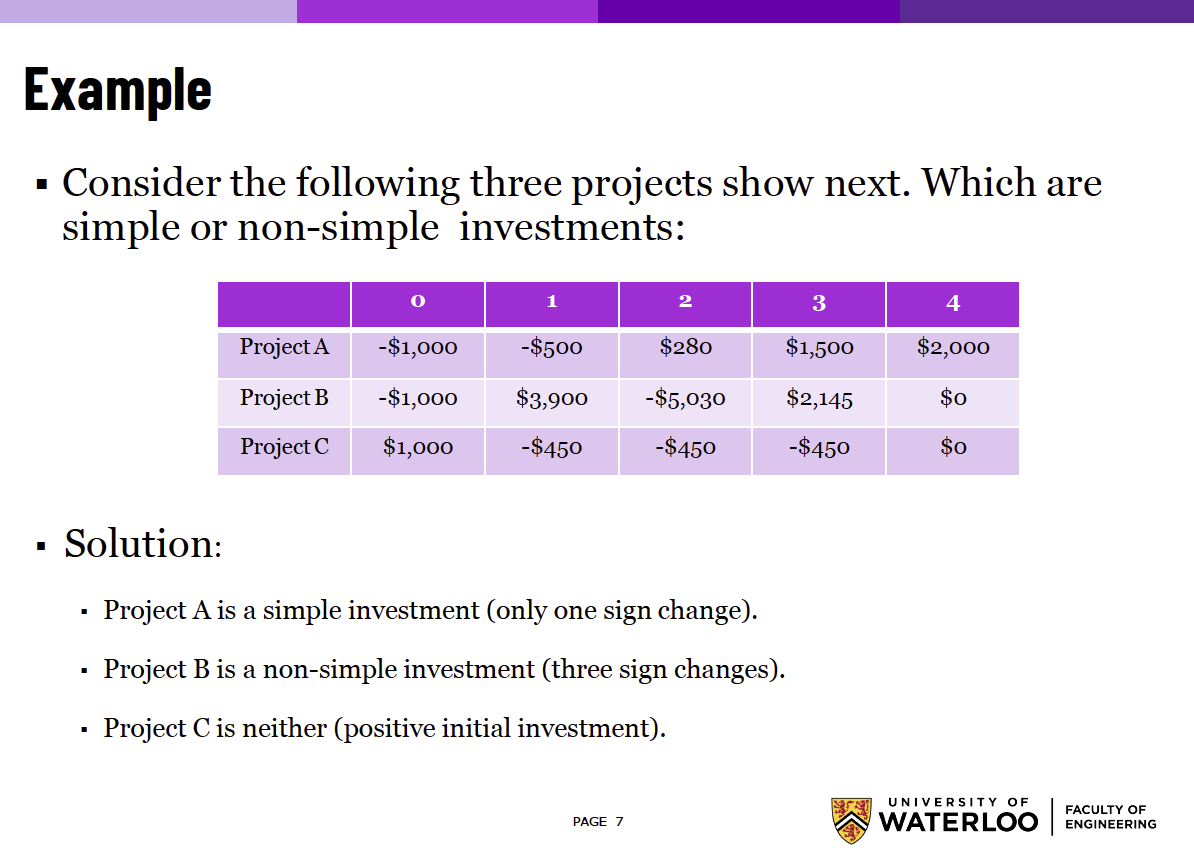# Rate of Return Analysis (IRR) §

Basically, we are solving for an unknown interest rate .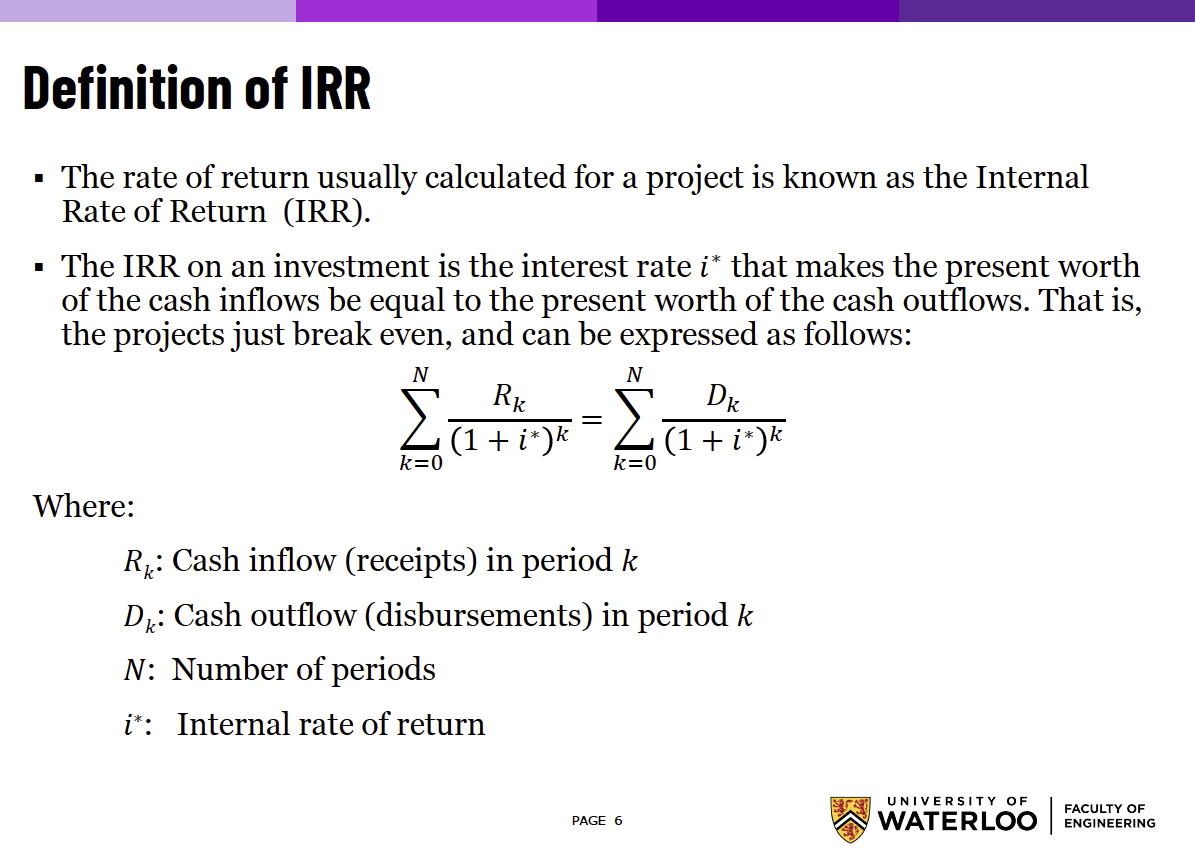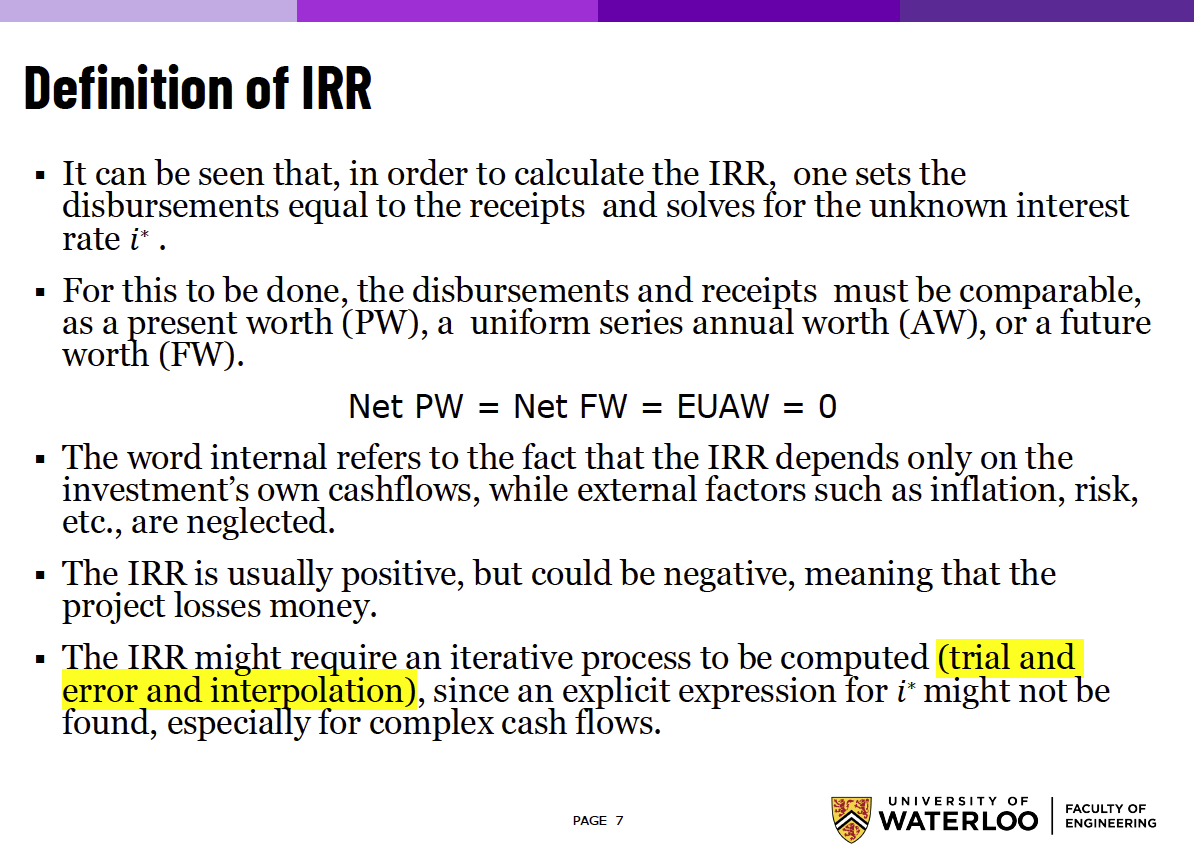• The idea when we solve is to set the Net Present Worth to 0, and solve for

#### Internal Rate of Return Analysis (IRR) §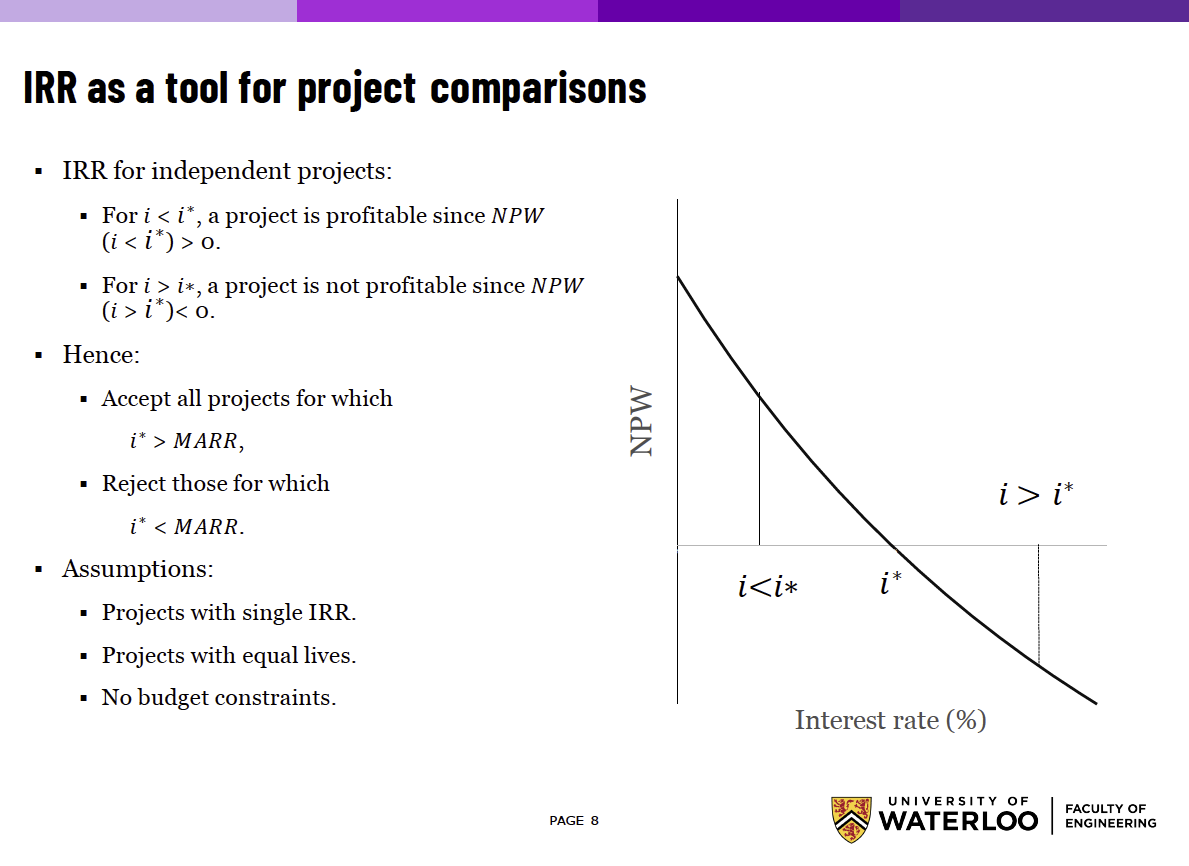The example below was so confusing to me, but I finally get it. They probably should have shown example 2 first though.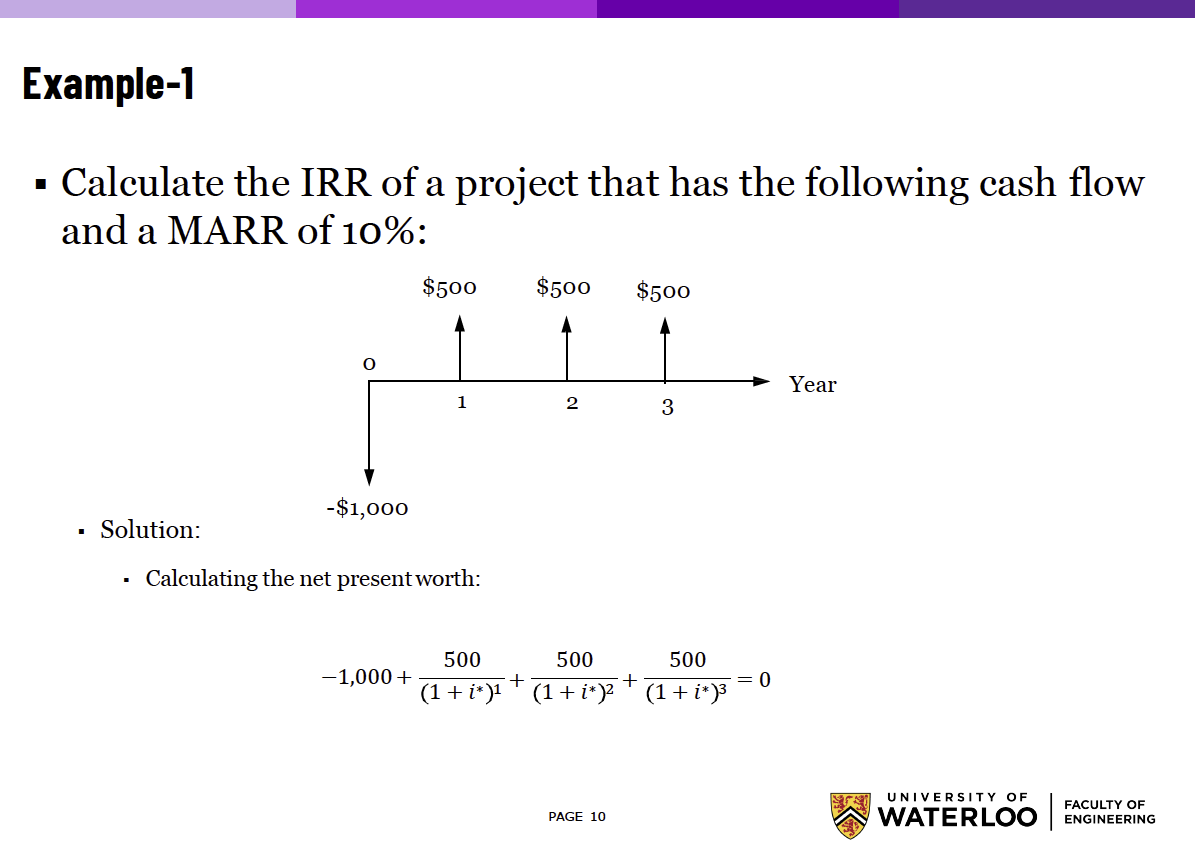• The idea is that we are trying to solve for . Below, they show a table with different values of , and the values that they return for the RHS.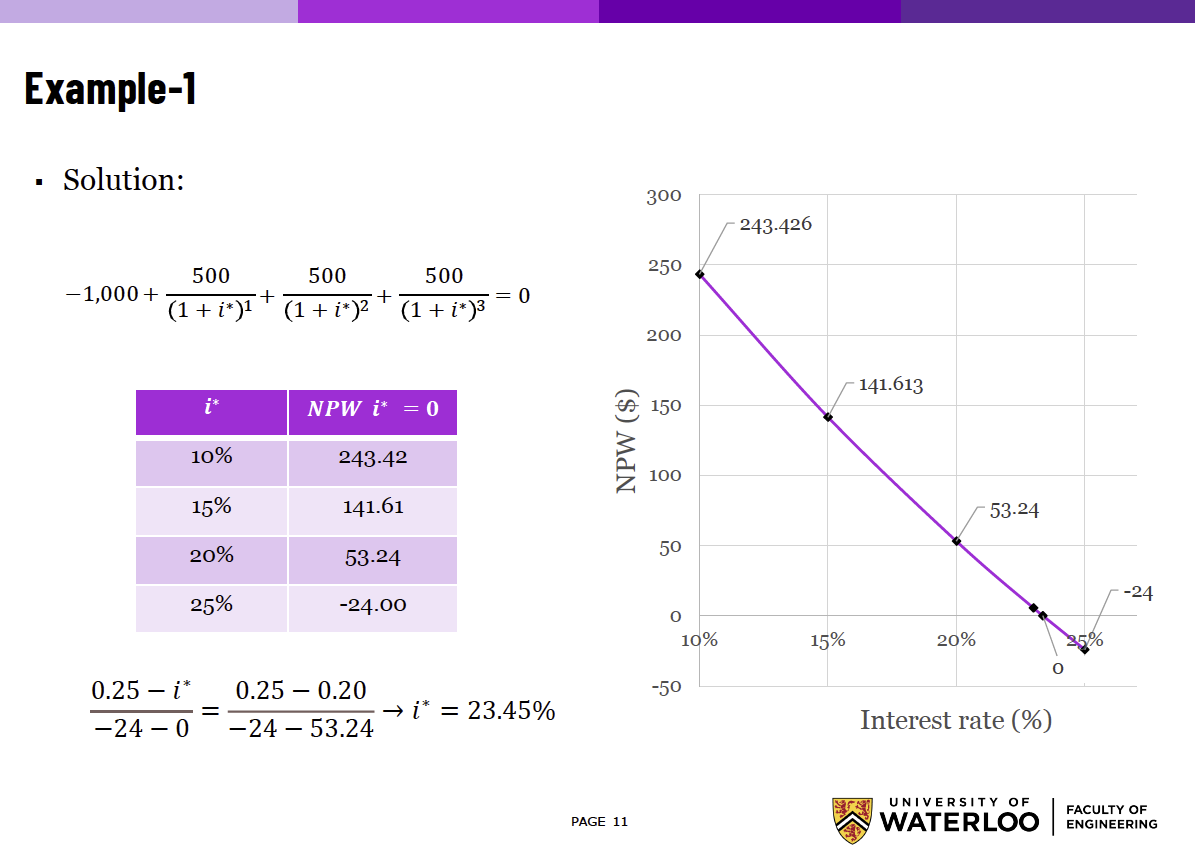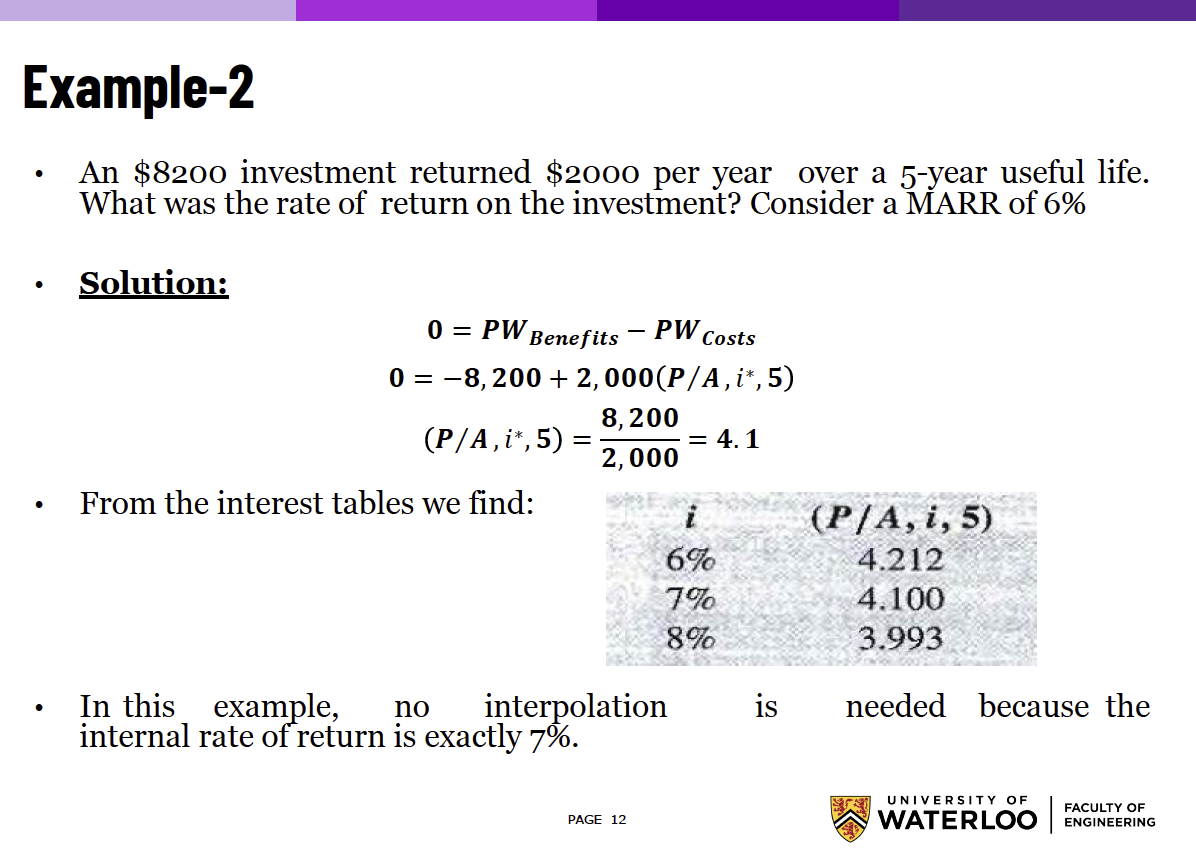#### Incremental Rate of Return (ΔIRR) §

ummm so do we always just use the incremental rate of return, or do we do the other things too?

IMPORTANT: To compare two mutually exclusive projects ( and ), we will write the cash flow of as .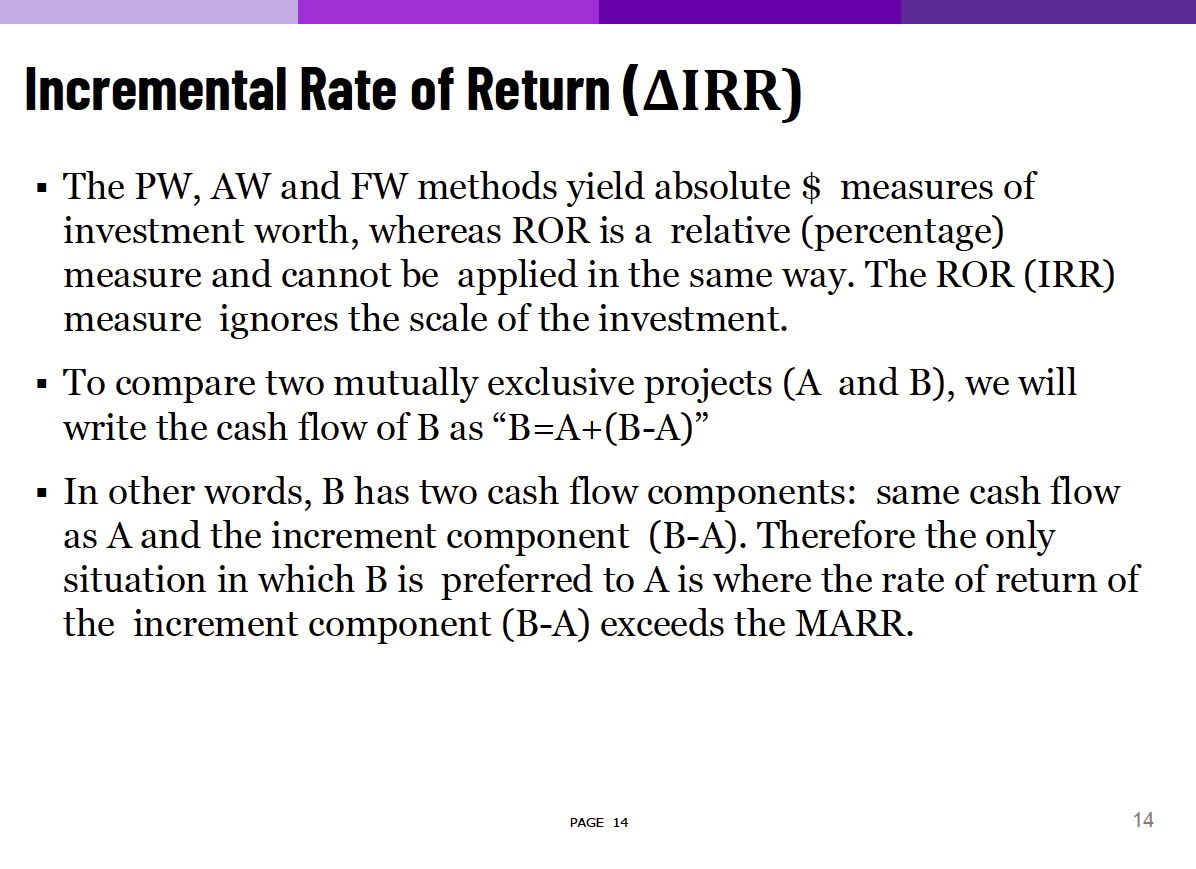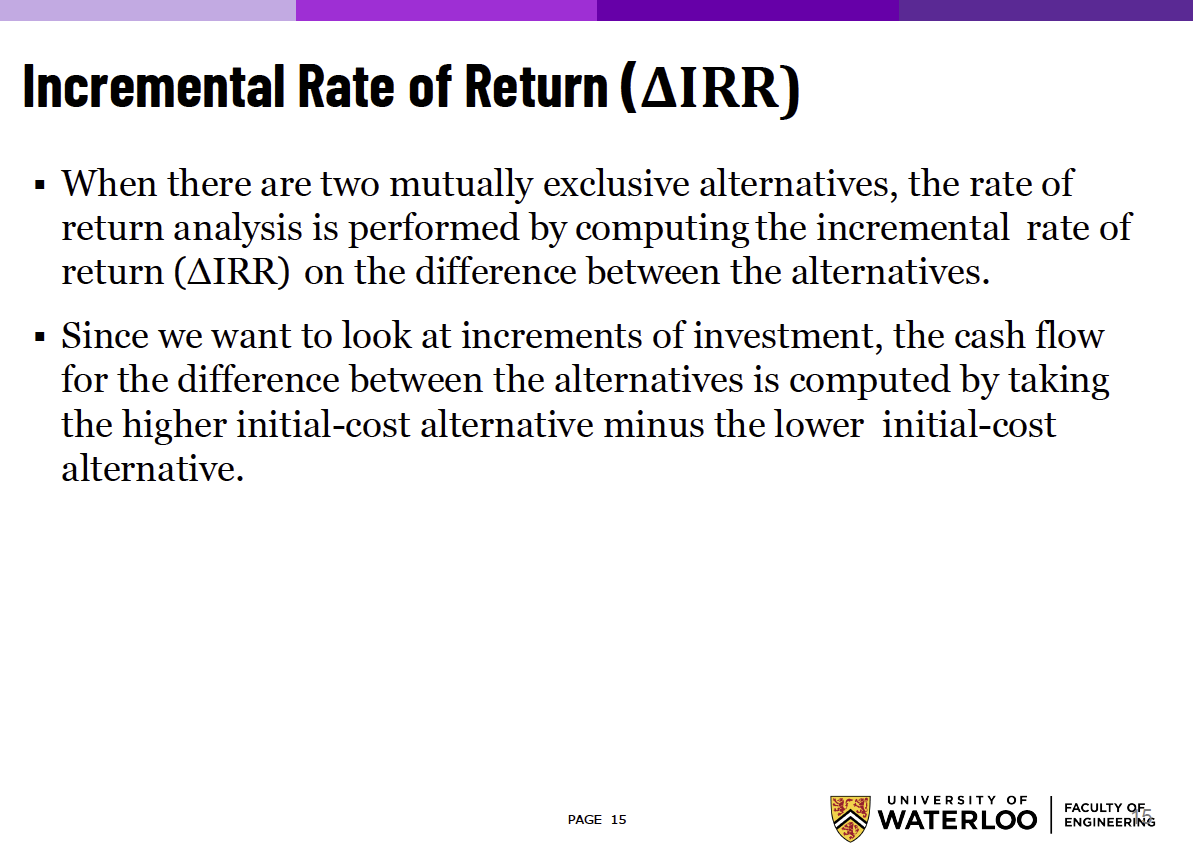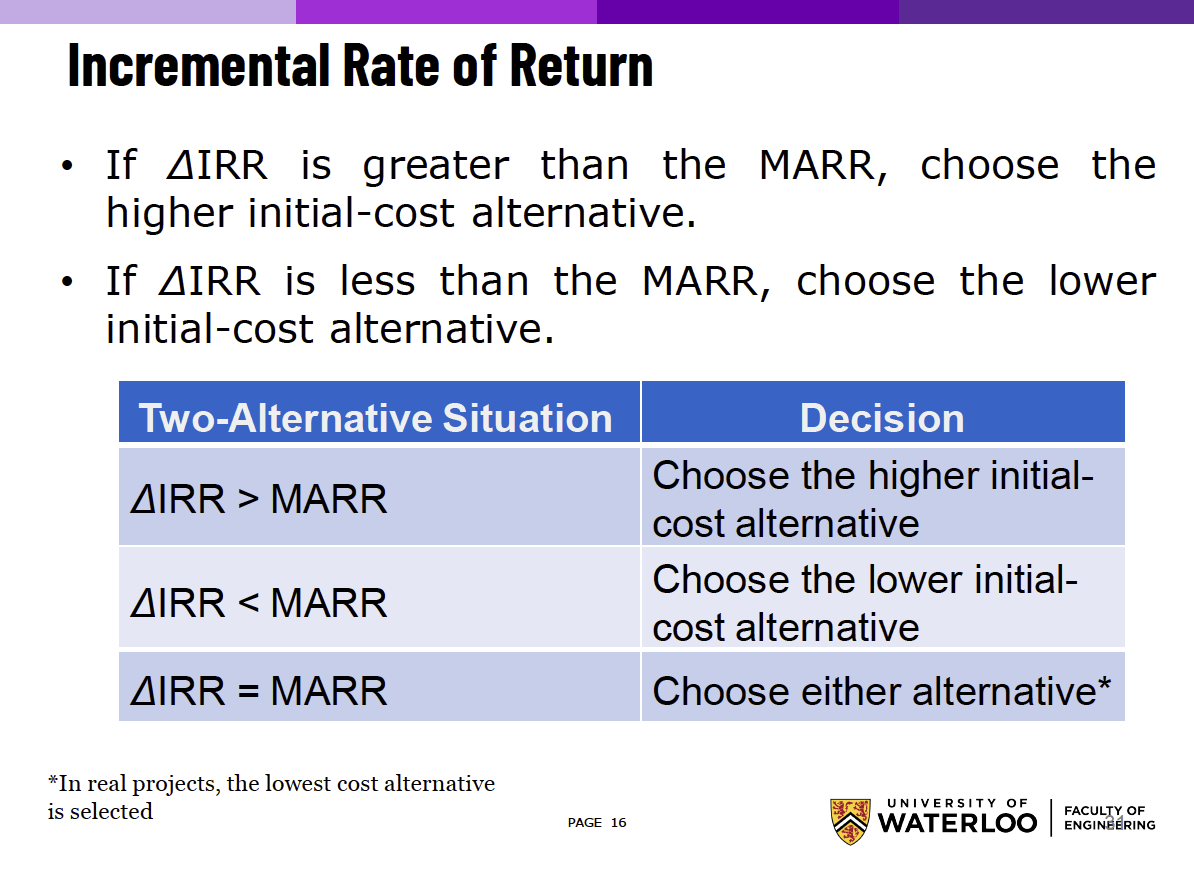This part seems way to complicated?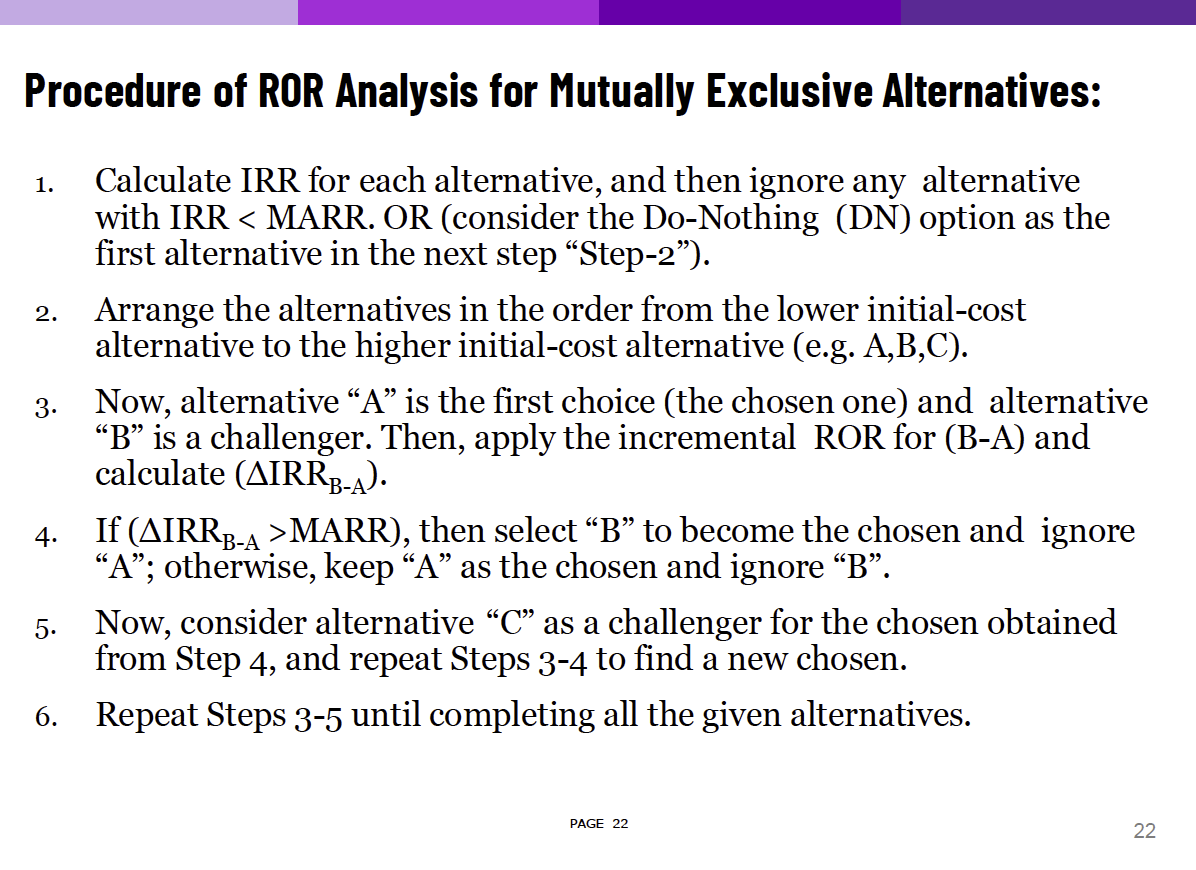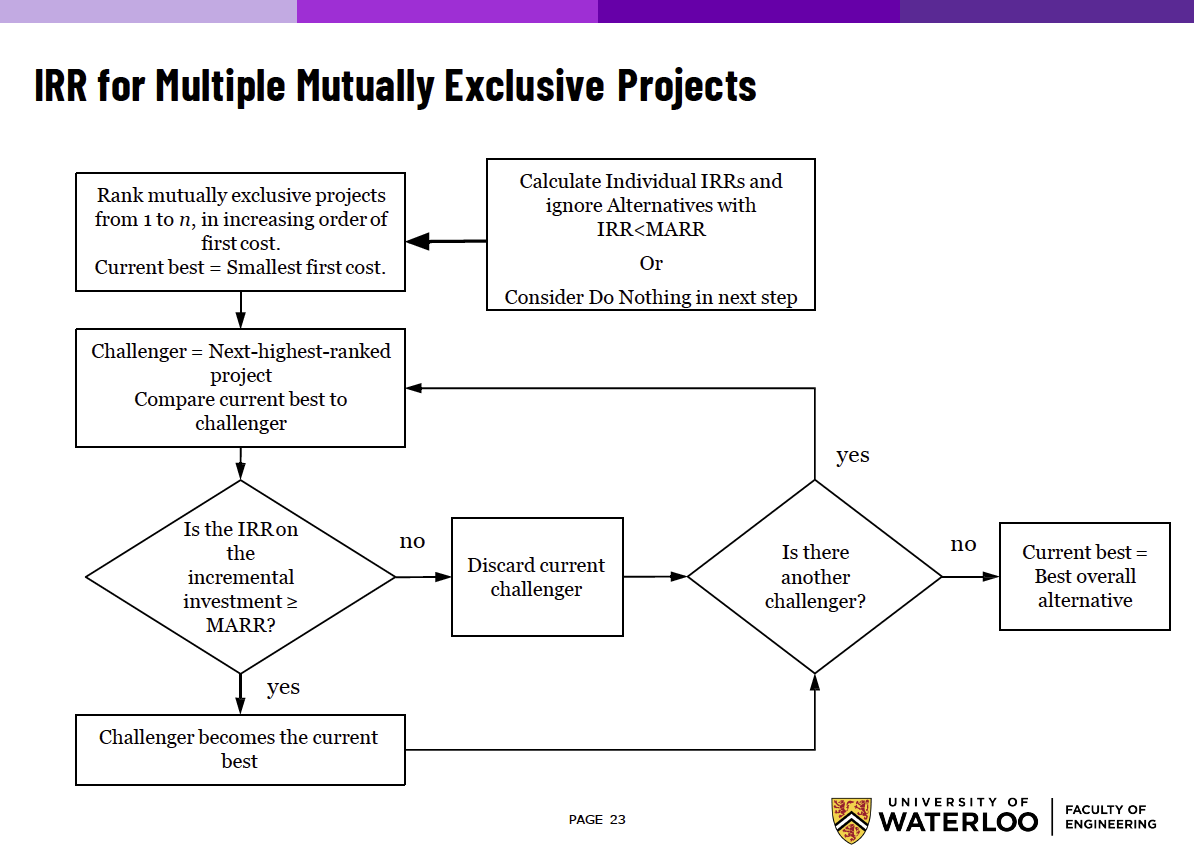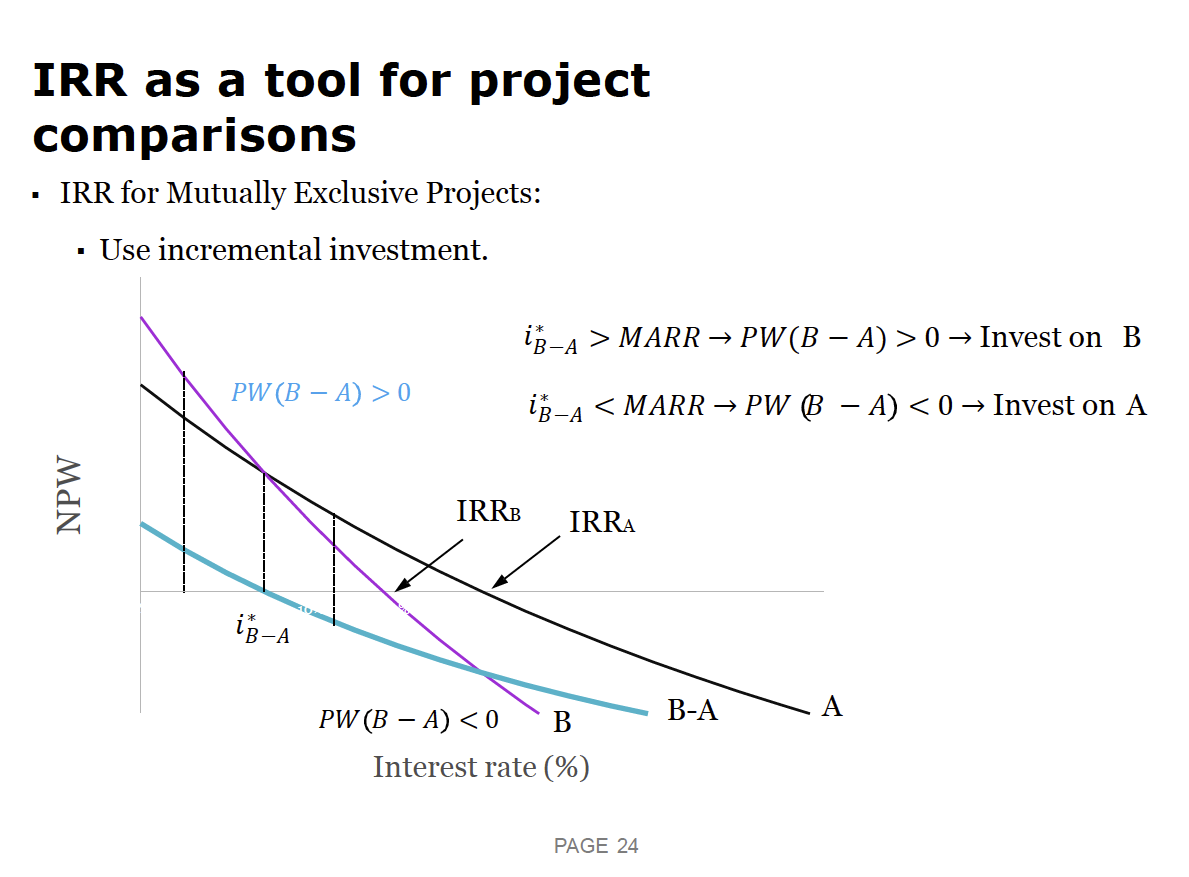• This diagram seems like a good explanation?
##### Examples §

They use a pretty drawn out explanation for this first example.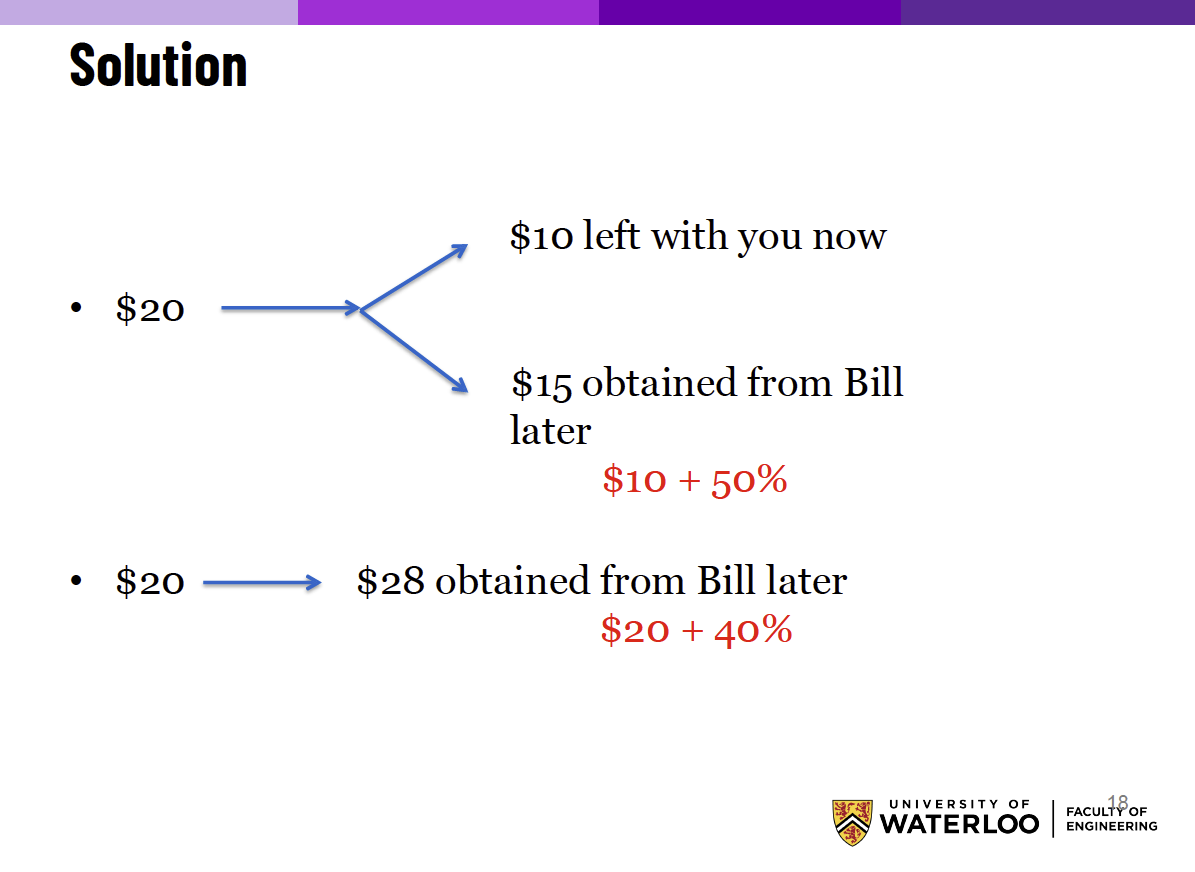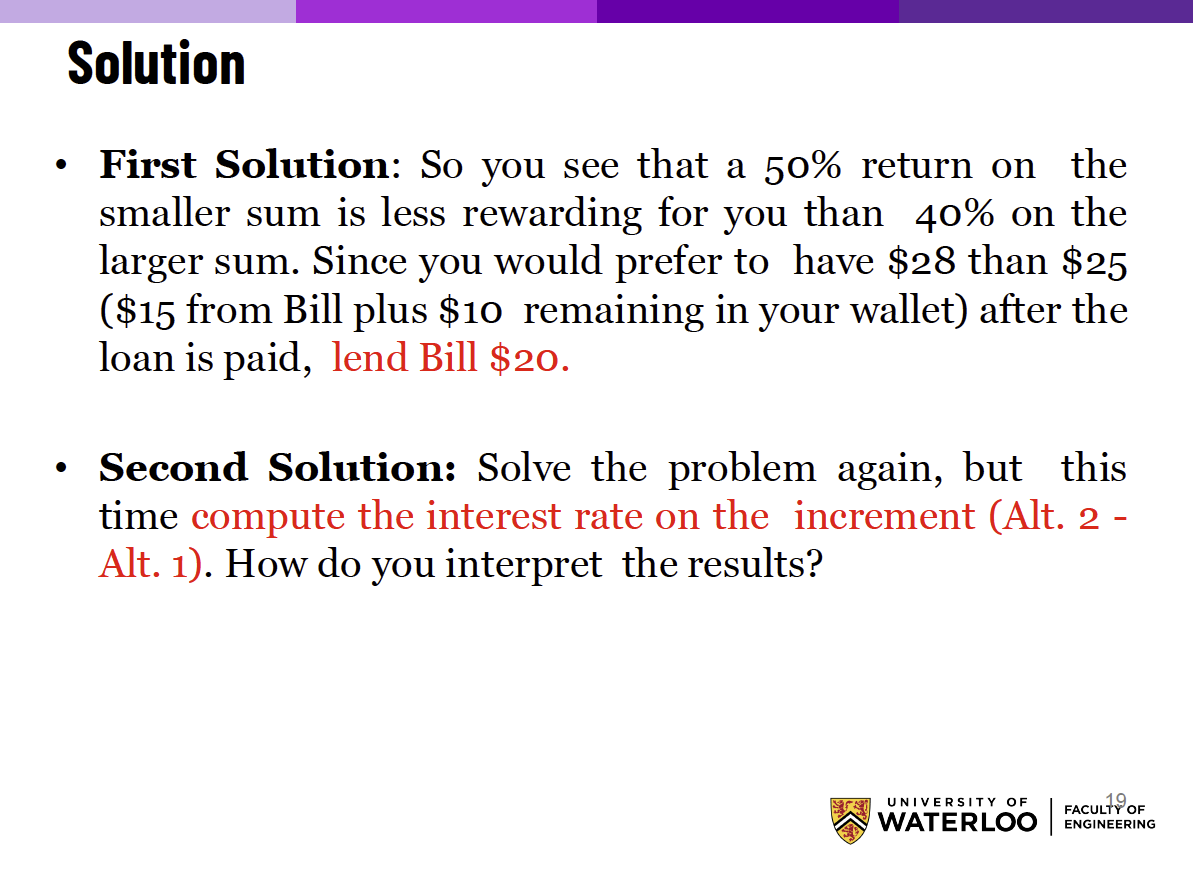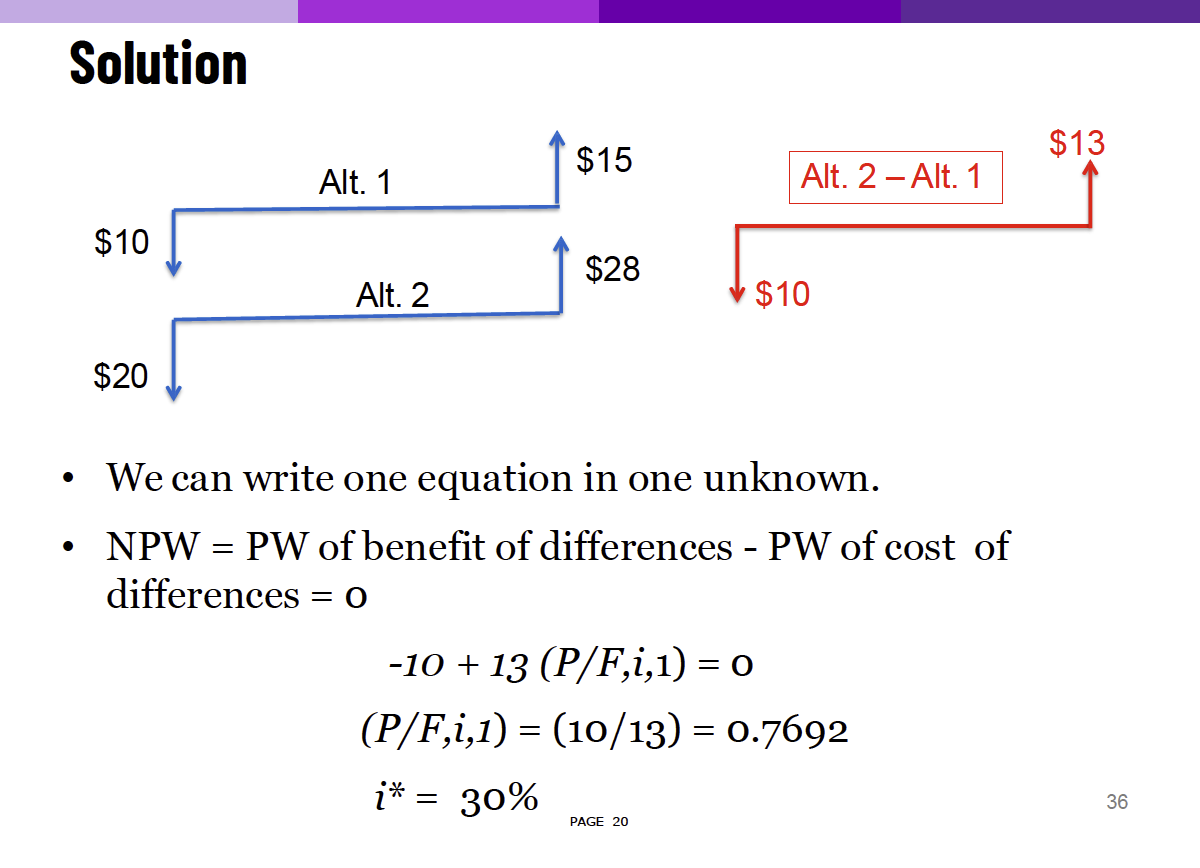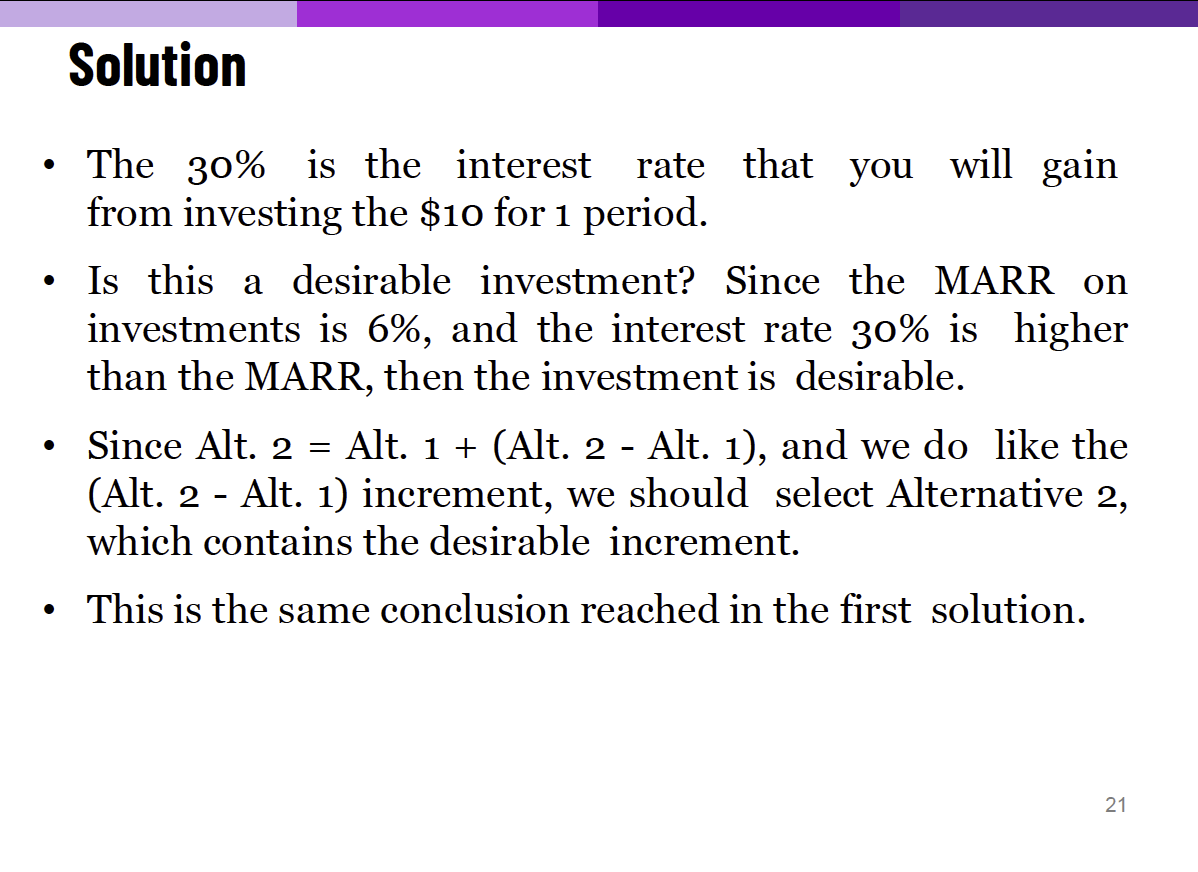So I think the template is just to set project 1 as the one with the cheaper initial cost. Then, you calculate 3 different IRR, and see which one surpasses the MARR to make your decision.

• Actually, you only need to calculate the third one:

“If the rate of return of the incremental investment is larger than the MARR, then alternative 2 should be selected; otherwise, alternative 1 must be chosen.”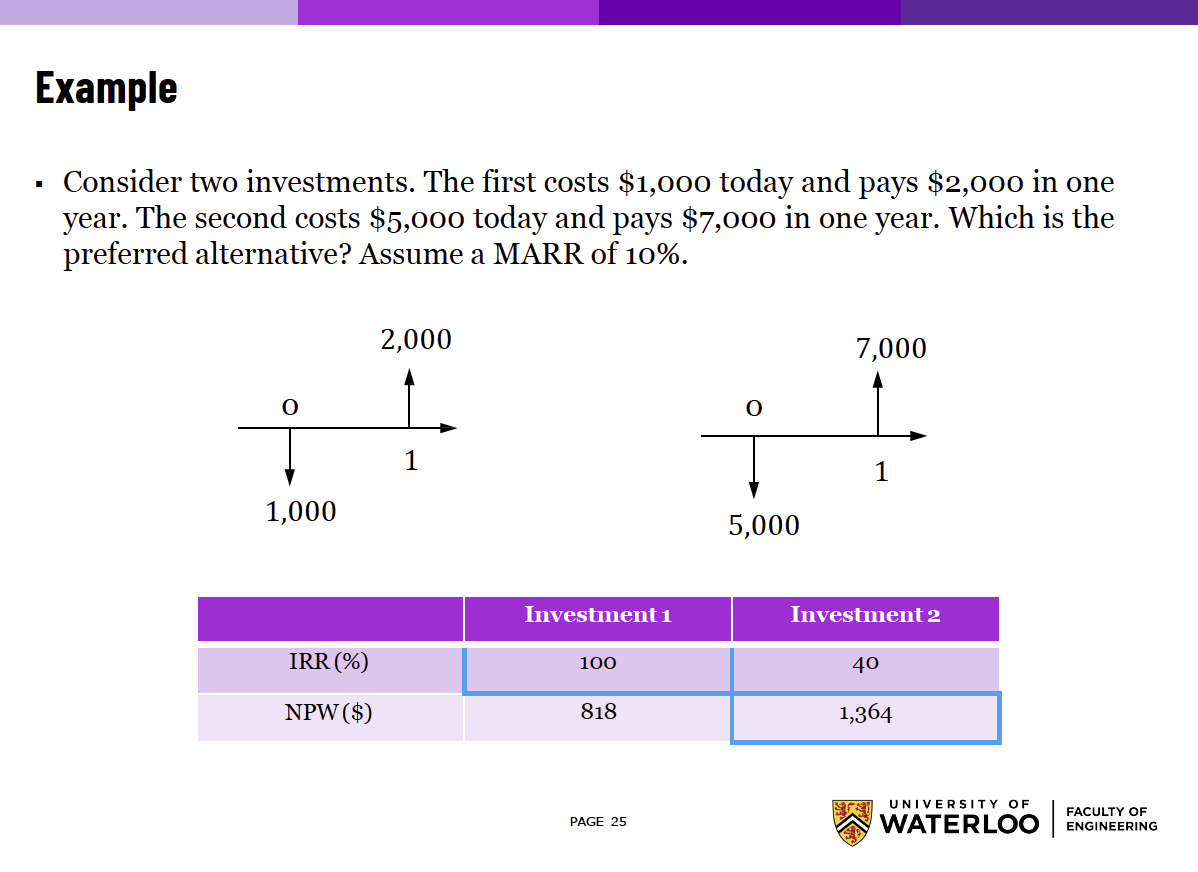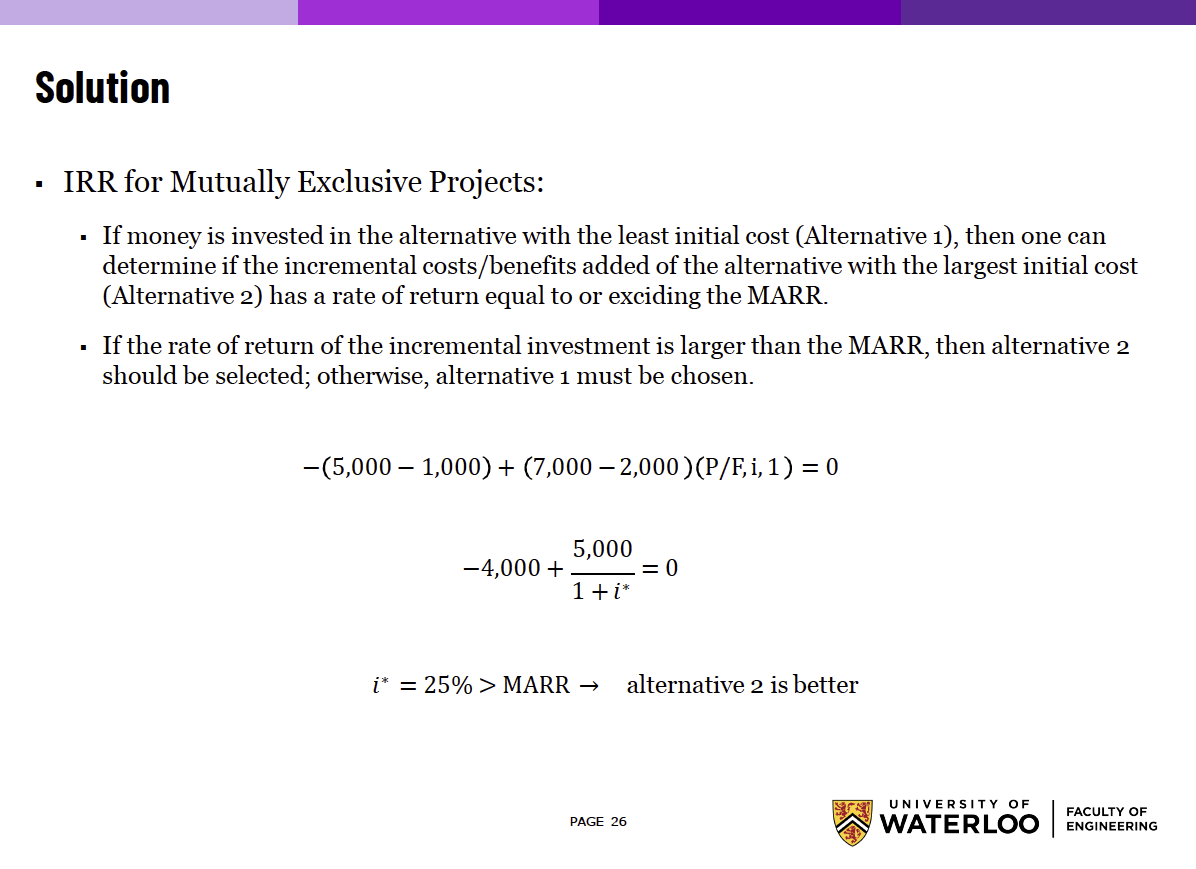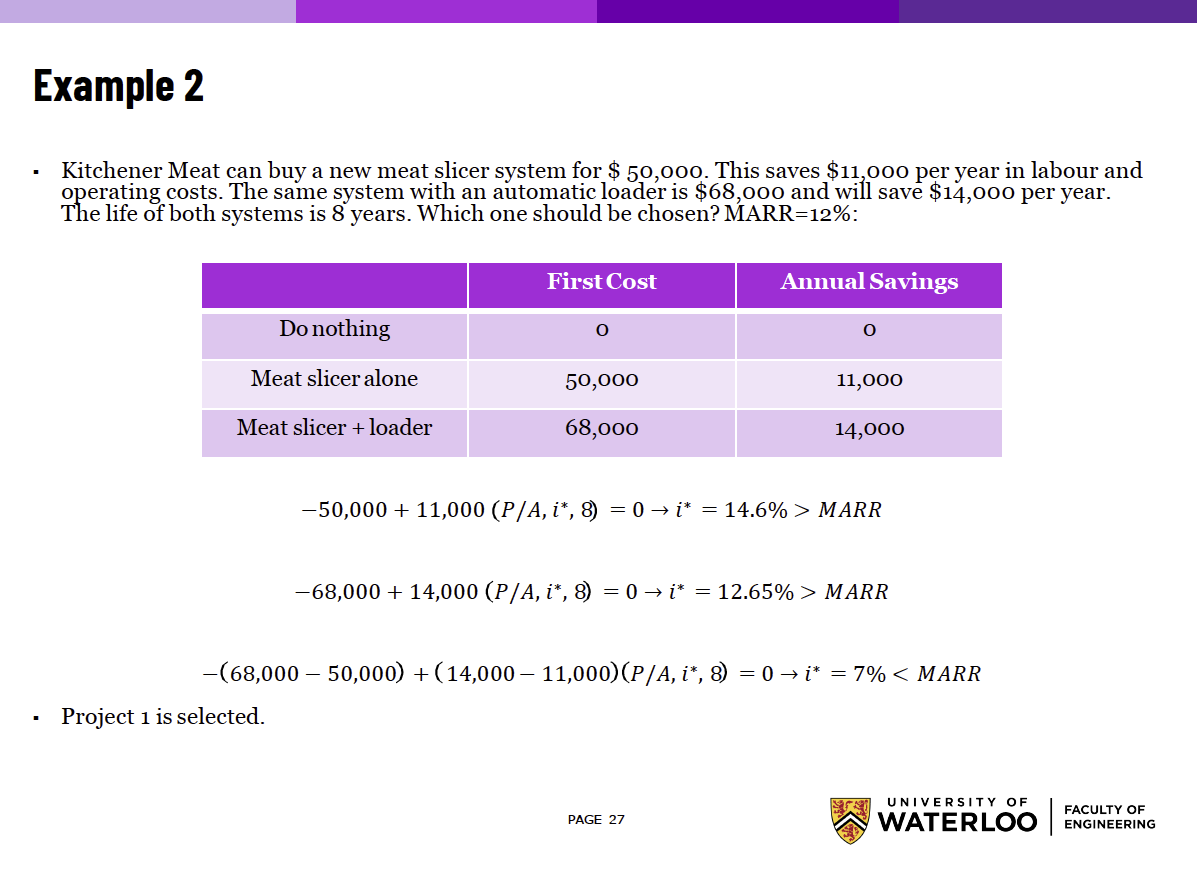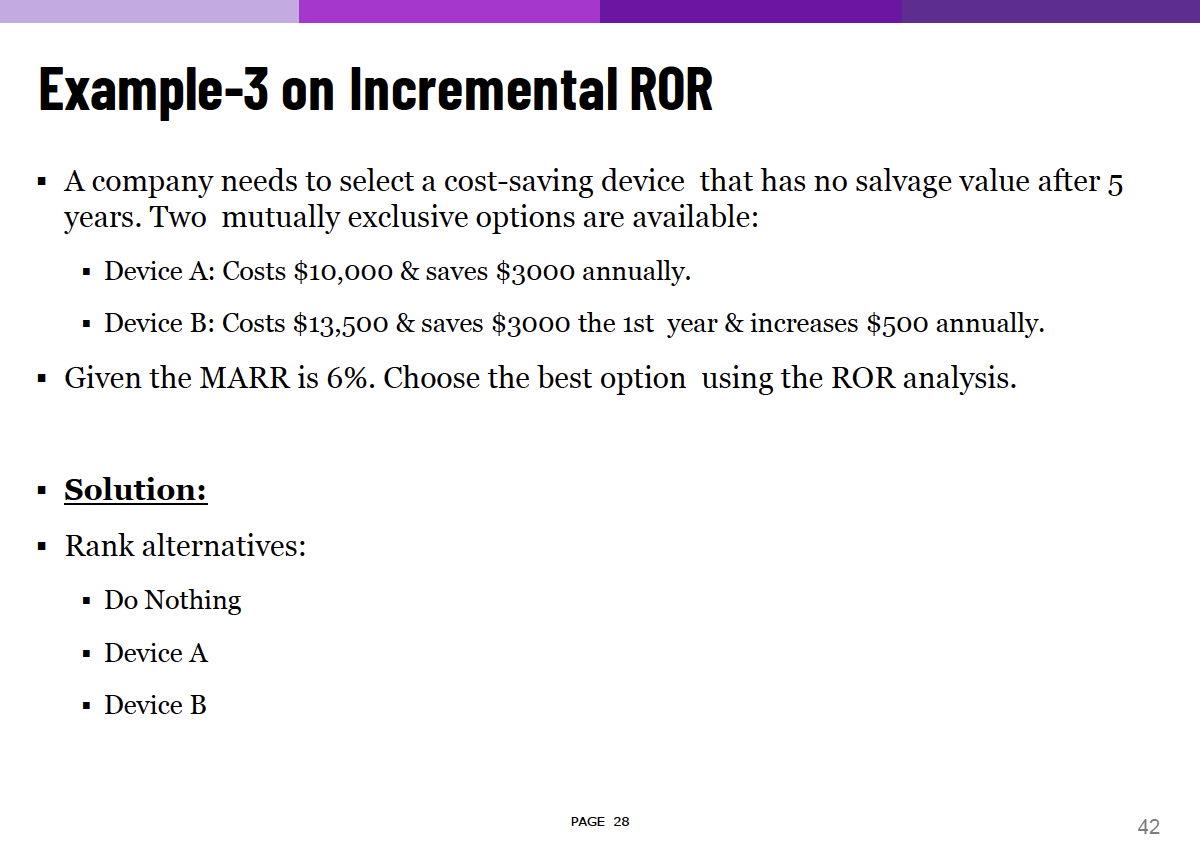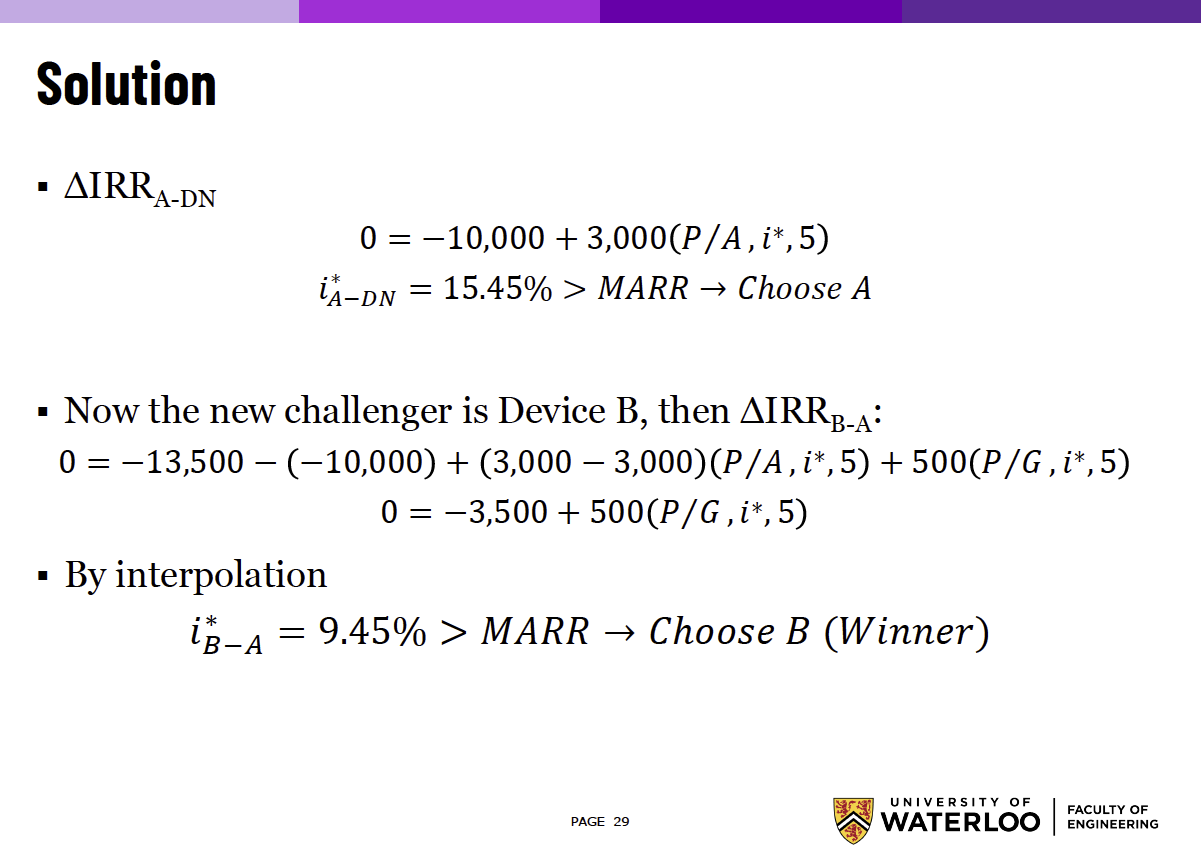#### Multiple IRR §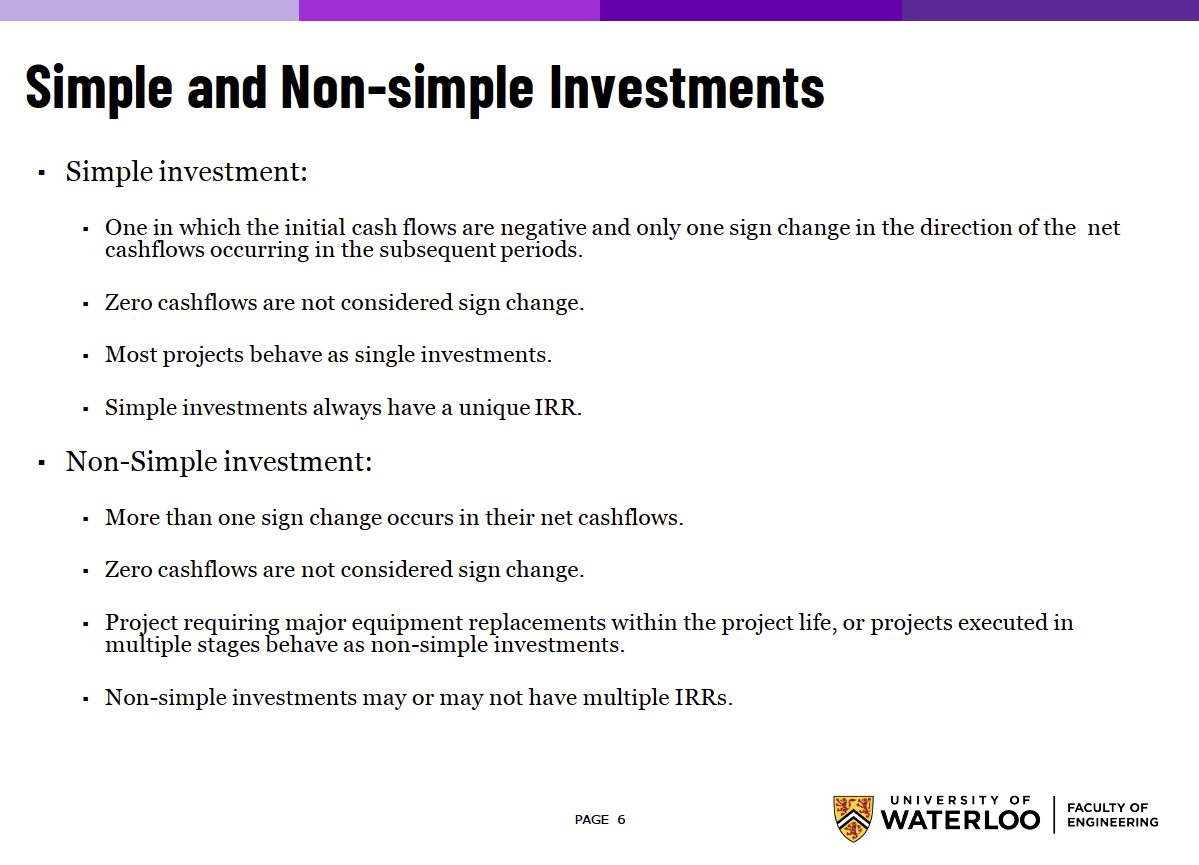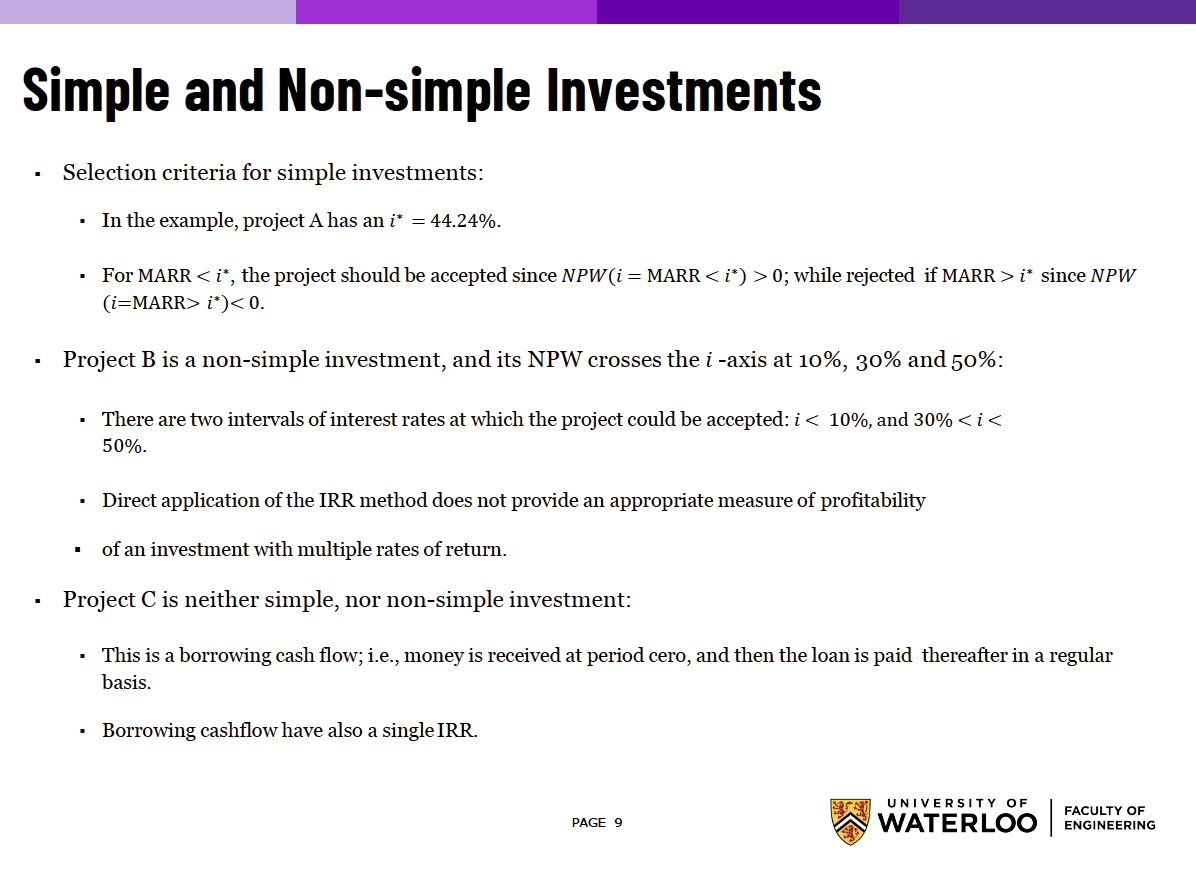Make sure to wrap your head around this: